• 《数据分析方法》–梅长林 各章原理及R语言实现 数据描述性分析 回归分析 方差分析 主成分分析典型相关分析 判别分析 聚类分析 Bayes统计分析 4.1主成分分析 4.1.1总体主成分的求法   求主成分归结为求样本...

《数据分析方法》–梅长林 各章原理及R语言实现

数据描述性分析
回归分析
方差分析
4. 主成分分析与典型相关分析
判别分析
聚类分析
Bayes统计分析

4.1主成分分析
4.1.1总体主成分的求法
求主成分归结为求样本（$X$）的协方差矩阵$\Sigma$的特征值和特征向量问题，具体由如下结论：
设$\Sigma$是$X=(X_1,X_2,...,X_p)^T$的而协方差矩阵，其特征值按大小顺序排列为$\lambda_1 \geq \lambda_2 \geq ...\geq \lambda_p \geq 0$，相应的正交单位化向量为 $e_1,e_2,...,e_p$，则$X$的第$k$个主成分可表示为
$Y_k = e_k ^ TX=e_{k1}X_1 + e_{k2}X_2 + ... + e_{kp}X_p, \quad k=1,2,...,p,$
其中$e_k=(e_{k1},e_{k2},...,e_{kp})^T$,并且我们知道$e_{k1}X_1 , e_{k2}X_2 , ... , e_{kp}X_p$相互独立，这时有：
$\begin{cases} &Var(Y_k) = e_k^T\Sigma e_k = \lambda_k e_k^Te_k = \lambda _k, \quad k=1,2,...,p,\\ &Cov(Y_j, Y_k) = e_j^T\Sigma e_k = \lambda_k e_j^Te_k = 0, \quad j\neq k, \end{cases}$
事实上，令$P=(e_{1},e_{2},...,e_{p})$，则$P$为正交矩阵，且
$P^T\Sigma P=\Lambda=Diag(\lambda_1 , \lambda_2 , ..., \lambda_p)，$
其中$Diag(\lambda_1 , \lambda_2 , ..., \lambda_p)$表示对角矩阵（因为协方差矩阵是实对称矩阵，所以可以正交对角化）。
若$Y_1=a_1^TX$为$X$的第一主成分，其中$a^T _ 1a_1=1$（约束条件：a的模为1，要不方差可以无穷大）令
$z_1=(z_{11},z_{12}, ...,z_{1p})^T=P^Ta_1$
则$z_1^Tz_1=a_1^TPP^Ta_1=a_1^Ta_1=1,且$
\begin{aligned} Var(Y_1)&=a_1^T\Sigma a_1 = z_1^TP^T\Sigma Pz_1\\&= \lambda_1z_{11} ^2 + \lambda_2 z_{12} ^ 2 + ... + \lambda_pz_{1p} ^ 2 \\& \leq \lambda_1z_1^Tz_1=\lambda_1 \end{aligned}
并且当$z_1=(1,0,..,0)^T$时，等号成立，这时
$a_1=Pz_1=e_1，$
由此可知，在约束条件$a_1^Ta_1=1$之下，当$a_1=e_1$时，$Var(Y_1)$达到最大，且
$\mathop{max}\limits_{a_1^T\Sigma a_1} \{Var(Y_1)\}=Var(e_1^TX)=e_1^T\Sigma e_1=\lambda_1$
设$Y_2=a_2^T$为$X$的第二主成分，则应有
$a_2^Ta_2=1且Cov(Y_2,Y_1)=a_2^T\Sigma e_1=\lambda_1a_2^Te_1=0,（Y_2、Y_1相互独立）$
即$a_2^Te_1=0.$令
$z_2=(z_{21},z_{22}, ...,z_{2p})^T=P^Ta_2$
则$z_2^Tz_2=1$，而由$a_2^Te_1=0$即有
$a_2^Te_1=z_2^TP^Te_1=z_{21}e_1^Te_1+z_{22}e_2^Te_1+...+z_{2p}e_p^Te_1=z_{21}=0$
故
\begin{aligned} Var(Y_2)&=a_2^T\Sigma a_2 = z_2^TP^T\Sigma Pz_2=z_2^T\Lambda z_2\\&= \lambda_1z_{21} ^2 + \lambda_{2}z_{22} ^ 2 + ... + \lambda_pz_{1p} ^ 2 \\&= \lambda_{2}z_{22} ^ 2 + ... + \lambda_pz_{1p} ^ 2 \leq \lambda_2 z_2^Tz_2 = \lambda_2 \end{aligned}
当$z_2=(0,1,0,...,0)^T$时，即$a_2=Pz_2=e_2$时，满足$a_2^Ta_2=1$,且$Cov(Y_2, Y_1)=\lambda_1a_2^Te_1=0$，并且使$Var(Y_2)=\lambda_2$达到最大
4.1.2总体主成分的性质
（1）主成分的协方差矩阵及总方差
（2）主成分的贡献率与累计贡献率
（3）主成分$Y_I$与$X_j$的相关系数 $Cov(X)=\Sigma=(\sigma_{ij})_{p\times p}$
4.1.3 R语言根据原理自己实现
求协方差矩阵
$\begin{bmatrix} 1 & -2 & 0\\ -2 & 5 & 0 \\ 0 & 0 & 2 \end{bmatrix}$
的各主成分
## 生成协方差矩阵
df = matrix(c(1, -2, 0, -2, 5, 0, 0, 0, 2), ncol=3)
df


A matrix: 3 × 3 of type dbl

1-20
-2 50
0 02

利用R语言的内置函数eigen求特征值特征向量
用法：
eigen(x, symmetric, only.values = FALSE, EISPACK = FALSE)

参数
说明

x
a numeric or complex matrix whose spectral decomposition is to be computed. Logical matrices are coerced to numeric.

symmetric
if TRUE, the matrix is assumed to be symmetric (or Hermitian if complex) and only its lower triangle (diagonal included) is used. If symmetric is not specified, isSymmetric(x) is used.

only.values
if TRUE, only the eigenvalues are computed and returned, otherwise both eigenvalues and eigenvectors are returned.

EISPACK
logical. Defunct and ignored.

结果有两个：

values：特征值
vectors：特征向量

## 特征向量
eigen(df)$vectors  A matrix: 3 × 3 of type dbl -0.382683400.9238795 0.923879500.3826834 0.000000010.0000000 ## 特征值 eigen(df)$values


5.8284271247461920.17157287525381
所以X的三个主成分为：
\begin{aligned} Y_1 &= e_1^TX = -0.383X_1+0.924X_2;\\ Y_2 &= e_2^TX = X_3;\\ Y_3 &= e_3^TX = 0.924X1+0.383X_2; \end{aligned}
由上述结果可知，第一主成分的贡献率为：
$\frac{5.83}{5.83 +2.00 + 0.17}=73%$
前两主成分的累计贡献率为：
$\frac{5.83+2.00}{5.83 +2.00 + 0.17}=989%$
因此，若用前两个主成分代替原来的三个变量，其信息损失仅为2%，是很小的。
4.1.4 R语言内置方法
R中作为主成分分析最主要的函数是 princomp() 函数

princomp() 主成分分析   可以从相关阵或者从协方差阵做主成分分析
summary() 提取主成分信息
predict() 预测主成分的值
screeplot() 画出主成分的碎石图
biplot() 画出数据关于主成分的散点图和原坐标在主成分下的方向

1.princomp()
## princomp()
df = data.frame(x1=rnorm(100), x2=rnorm(100), x3=rnorm(100))
df.pr=princomp(df,cor=FALSE) # cor=TRUE：以X的相关系数矩阵做主成分分析，其实就是X的标准化变量的主成分
df.pr

Call:
princomp(x = df, cor = FALSE)

Standard deviations:
Comp.1    Comp.2    Comp.3
1.0784416 0.9679190 0.8492227

3  variables and  100 observations.

其中Standard deviations代表各主成分的标准差，即根号特征值（特征值是主成分的方差）
2.summary()
summary(df.pr,loadings=TRUE)

Importance of components:
Comp.1    Comp.2    Comp.3
Standard deviation     1.078442 0.9679190 0.8492227
Proportion of Variance 0.412266 0.3320949 0.2556392
Cumulative Proportion  0.412266 0.7443608 1.0000000

Comp.1 Comp.2 Comp.3
x1         0.998
x2 -0.120        -0.991
x3  0.992        -0.121

其中Proportion of Variance是各主成分的贡献率
Cumulative Proportion是累计贡献率
3.predict()
predict(df.pr, data.frame(x1=c(1, 2), x2=c(3, 4), x3=c(4, 5))) # 预测自给的数据
#predirc(df.pr) # 预测已有数据的主成分


A matrix: 2 × 3 of type dbl

Comp.1Comp.2Comp.3

3.7700270.9634912-3.532819
4.6717941.9991897-4.588350

loadings(df.pr)

Loadings:
Comp.1 Comp.2 Comp.3
x1         0.998
x2 -0.120        -0.991
x3  0.992        -0.121

Comp.1 Comp.2 Comp.3
Proportion Var  0.333  0.333  0.333
Cumulative Var  0.333  0.667  1.000

5.screeplot()
## 条形碎石图
screeplot(df.pr)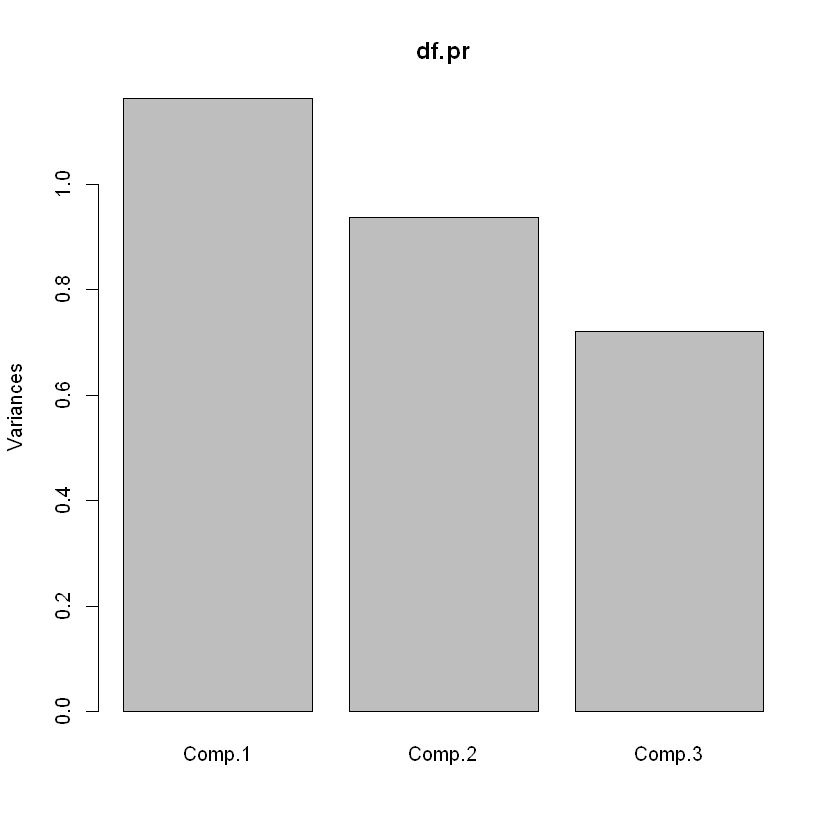## 折现碎石图
screeplot(df.pr, type='lines')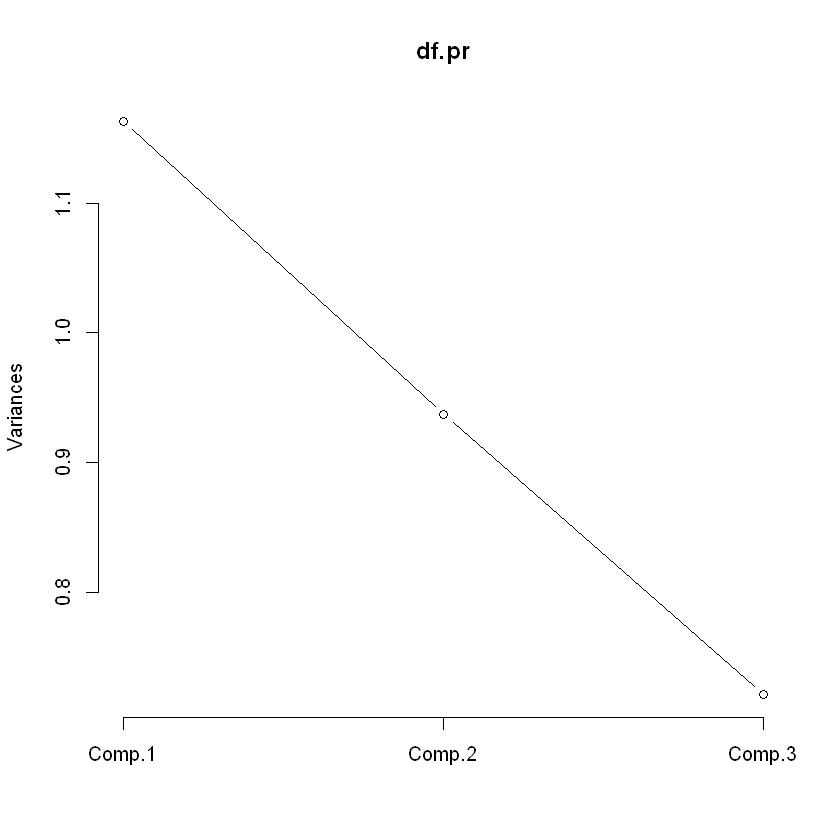6.biplot()
## 主成分散点图
biplot(df.pr)


[Image_Link]https://img-blog.csdnimg.cn/20200508204916838.png

4.2 典型相关分析
## 相关系数矩阵
CorX = matrix(c(1, 0.571, 0.875, 0.571, 1, 0.781, 0.875, 0.781, 1), ncol=3)
CorY = matrix(c(1, 0.671, 0.785, 0.671, 1, 0.932, 0.785, 0.932, 1), ncol=3)
CorXY = matrix(c(0.714, 0.840, 0.914, 0.380, 0.681, 0.591, 0.626, 0.819, 0.870), ncol=3)
CorYX = t(CorXY)

# 求负二次幂
fuermi<-function(Sigma){
lamda <- solve(eigen(Sigma)$vectors)%*%Sigma%*%(eigen(Sigma)$vectors)
sqrt(diag(lamda))
lamda_sqrt <- matrix(c(sqrt(diag(lamda)),0,0,0,sqrt(diag(lamda)),0, 0, 0, sqrt(diag(lamda))),nrow = 3,ncol = 3)
Sigma_sqrt <- (eigen(Sigma)$vectors)%*%lamda_sqrt%*%solve(eigen(Sigma)$vectors)
return(solve(Sigma_sqrt))
}

A2 = fuermi(CorX) %*% CorXY %*% solve(CorY) %*% CorYX %*% fuermi(CorX)
B2 = fuermi(CorY) %*% CorYX %*% solve(CorX) %*% CorXY %*% fuermi(CorY)

e1 = eigen(A2)$vectors e2 = eigen(B2)$vectors

rho1 = eigen(B2)$values rho2 = eigen(B2)$values
rho3 = eigen(B2)$values  # 典型相关系数 c(rho1, rho2, rho3)  1.21168515342770.2299340139989880.0274354332393604 ## X的典型相关变量系数 e1  A matrix: 3 × 3 of type dbl -0.3287070 0.2380847 0.9139296 -0.3796076-0.9193985 0.1029784 -0.8647831 0.3130849-0.3925915 ## Y的典型相关变量系数 e2  A matrix: 3 × 3 of type dbl -0.60894743-0.2898345 0.7383624 0.04600424-0.9421908-0.3319037 -0.79187539 0.1681441-0.5870783 希望我的文章对您有所帮助，同时也感谢您能抽出宝贵的时间阅读，打公式不易，如果您喜欢的话，欢迎点赞、关注、收藏。您的支持是我创作的动力，希望今后能带给大家更多优质的文章  展开全文• （可以看出与自相关的公式差不多，其实互相关分析就是两个时间序列在不同时刻暴力套上自相关系数的公式…大概可以这么理解） 在r中用ccf函数可以计算 这里以r中自带的airmiles数据集和LakeHuron（1937-1960年）进行... 互相关分析 互相关与自相关不同，互相关是指两个时间序列在任意两个不同时刻的相关程度。 假设有时间序列 xt 和 yt ，则 xt 在时刻 t 和 yt 在时刻 t+n 的相关即为n阶互相关。 公式：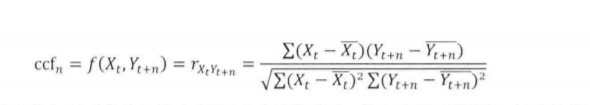重温一下自相关系数的公式：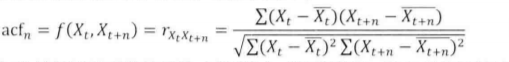（可以看出与自相关的公式差不多，其实互相关分析就是两个时间序列在不同时刻暴力套上自相关系数的公式…大概可以这么理解） 在r中用ccf函数可以计算 这里以r中自带的airmiles数据集和LakeHuron（1937-1960年）进行演示。 ccf(airmiles,ts(LakeHuron,start = 1937,end = 1960))  （ts函数可以选择数据集中的一部分）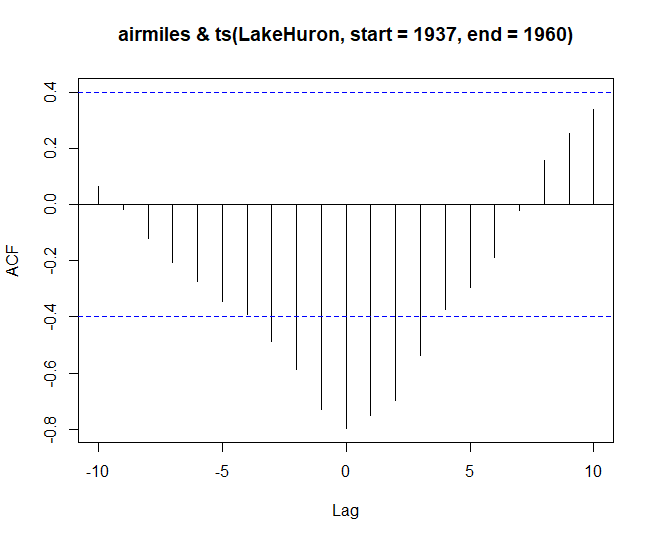可以看出在没有延迟（lag=0）的时候，互相关系数为-0.8达到了最小，此时呈负相关。当延迟为10时，呈若正相关，因此可以通过互相关性的分析。 典型相关分析 典型相关分析是指两组变量间的相关关系（这里的相关不像之前的几种相关，变量两两组合求相关系数），而是反应两个整体（两组变量）之间的相关性。其难点是如何构建综合指标使两组数据的相关性最大 这里跳过理论，r语言中stats包中的cancor函数可直接计算典型相关系数。 现使用iris数据集，分别将第一、二列，第三、四列转化为数值矩阵 x<-as.matrix(iris[,1:2]) y<-as.matrix(iris[,3:4])  再使用cancor函数求解 x 与 y 的典型相关系数 > cancor(x,y)$cor
 0.9409690 0.1239369

$xcoef [,1] [,2] Sepal.Length -0.08757435 0.04749411 Sepal.Width 0.07004363 0.17582970$ycoef
[,1]       [,2]
Petal.Length -0.06956302 -0.1571867
Petal.Width   0.05683849  0.3940121

$xcenter Sepal.Length Sepal.Width 5.843333 3.057333$ycenter
Petal.Length  Petal.Width
3.758000     1.199333

代码运行结果可看出，典型相关系数有两个， 0.9409690 和 0.1239369。
第一组变量的典型相关系数较强，第二组较弱，说明第一组适合做分析。
小结
互相关分析与典型相关分析都是分析两个不同的数据集之间的相关性。互相关分析的对象为时间序列中两个不同时刻的相关程度，典型相关分析的对象为两个数据集之间的相关性


展开全文数据建模
• ## R语言典型相关分析

万次阅读 多人点赞 2017-02-27 16:40:46
部分参考薛毅的《统计建模与R软件》和《R语言实战》1 关键点：典型相关分析典型相关分析是用于分析两组随机变量之间的相关程度的一种统计方法，它能够有效地揭示两组随机变量之间的相互（线性依赖）关系例如 研究生...
自己整理编写的R语言常用数据分析模型的模板，原文件为Rmd格式，直接复制粘贴过来，作为个人学习笔记保存和分享。部分参考薛毅的《统计建模与R软件》和《R语言实战》

1 关键点：典型相关分析

典型相关分析是用于分析两组随机变量之间的相关程度的一种统计方法，它能够有效地揭示两组随机变量之间的相互（线性依赖）关系

例如 研究生入学考试成绩与本科阶段一些主要课程成绩的相关性

将研究两组变量的相关性问题转化为研究两个变量的相关性问题 此类相关为典型相关#

2 分类：

总体典型相关

样本典型相关

3 R语言提供的计算函数：

典型相关计算 cancor(x,y,xcenter=TRUE,ycenter=TRUE)

x,y是相应的数据矩阵 xcenter，ycenter是逻辑变量 TRUE是将数据中心化 FALSE是不中心化

4 分析结果含义

cor是典型相关系数

xcoef是对应于数据x的系数 又称关于数据x的典型载荷即样本典型变量U系数矩阵A的转置

xcenter是数据X的中心 即数据X的样本均值

y是对应于数据x的系数 又称关于数据y的典型载荷即样本典型变量V系数矩阵B的转置

ycenter是数据Y的中心 即数据Y的样本均值

5 分析步骤

（1.）载入原始数据  data.frame

（2.）原始数据标准化  scale

（3.）典型相关分析   cancor

（4.）相关系数显著性检验  corcoef.test.R

I.典型相关分析的计算

现对20名中年人测得三个生理指标：体重(X1) 腰围(X2) 脉搏(X3);三个训练指标:引体向上(Y1) 起座次数(Y2)  跳跃次数(Y3)  试分析这组数据的相关性

#用数据框的形式输入数据矩阵
test<-data.frame(
X1=c(191, 193, 189, 211, 176, 169, 154, 193, 176, 156,
189, 162, 182, 167, 154, 166, 247, 202, 157, 138),
X2=c(36, 38, 35, 38, 31, 34, 34, 36, 37, 33,
37, 35, 36, 34, 33, 33, 46, 37, 32, 33),
X3=c(50, 58, 46, 56, 74, 50, 64, 46, 54, 54,
52, 62, 56, 60, 56, 52, 50, 62, 52, 68),
Y1=c( 5, 12, 13,  8, 15, 17, 14,  6,  4, 15,
2, 12,  4,  6, 17, 13,  1, 12, 11,  2),
Y2=c(162, 101, 155, 101, 200, 120, 215,  70,  60, 225,
110, 105, 101, 125, 251, 210,  50, 210, 230, 110),
Y3=c(60, 101, 58, 38, 40, 38, 105, 31, 25, 73,
60, 37, 42, 40, 250, 115, 50, 120, 80, 43)
)
#为了消除数量级的影响 将数据标准化处理 调用scale函数
test<-scale(test)
#对标准化的数据做典型相关分析
ca<-cancor(test[,1:3],test[,4:6])
#查看分析结果
ca

结果说明:
1) cor给出了典型相关系数;<!--//--><![CDATA[//><!--
cor给出了典型相关系数;
//--><!]]>xcoef是对应于数据X的系数, 即为关于数据X的典型载荷; ycoef为关于数据Y的典型载荷;<!--//--><![CDATA[//><!--
ycoef为关于数据Y的典型载荷;
//--><!]]>xcenter与$ycenter是数据X与Y的中心, 即样本均值; 2) 对于该问题, 第一对典型变量的表达式为 U1 = -0.17788841x1 + 0.36232695x2 - 0.01356309x3 U2 = -0.43230348x1 + 0.27085764x2 - 0.05301954x3 U3 = -0.04381432x1 + 0.11608883x2 + 0.24106633x3 V1 = -0.08018009y1 - 0.24180670y2 + 0.16435956y3 V2 = -0.08615561y1 + 0.02833066y2 + 0.24367781y3 V3 = -0.29745900y1 + 0.28373986y2 - 0.09608099y3 相应的相关系数为：p(U1,V1)=0.79560815 ,p(U2,V2)=0.20055604 ,p(U3,V3)=0.07257029 可以进行典型相关系数的显著性检验, 经检验也只有第一组典型变量. 下面计算样本数据在典型变量下的得分： #计算数据在典型变量下的得分 U=AX V=BY U<-as.matrix(test[, 1:3])%*% ca$xcoef ; U
V<-as.matrix(test[, 4:6])%*% ca$ycoef ; V #调整图形 opar <- par(mfrow = c(1, 1),mar = c(5,4,1,1)) #画出以相关变量U1、V1和U3、V3为坐标的数据散点图 plot(U[,1], V[,1], xlab="U1", ylab="V1") plot(U[,3], V[,3], xlab="U3", ylab="V3") #调整图形 par(opar) 由散点图可知 第一典型相关变量分布在一条直线附近；第三典型相关变量数据很分散。因为第一典型变量其相关系数为0.79560815，接近1，所以在一直线附近；第三典型变量的相关系数是0.07257029，接近于0，所以很分散。 II.典型相关系数的显著性检验 作为相关分析的目的 就是选择多少对典型变量？因此需要做典型相关系数的显著性检验。若认为相关系数k为0 就没有必要考虑第k对典型变量了 #相关系数检验R程序 corcoef.test<-function(r, n, p, q, alpha=0.1){ #r为相关系数 n为样本个数 且n>p+q m<-length(r); Q<-rep(0, m); lambda <- 1 for (k in m:1){ #检验统计量 lambda<-lambda*(1-r[k]^2); #检验统计量取对数 Q[k]<- -log(lambda) } s<-0; i<-m for (k in 1:m){ #统计量 Q[k]<- (n-k+1-1/2*(p+q+3)+s)*Q[k] chi<-1-pchisq(Q[k], (p-k+1)*(q-k+1)) if (chi>alpha){ i<-k-1; break } s<-s+1/r[k]^2 } #显示输出结果 选用第几对典型变量 i } source("corcoef.test.R") #输入相关系数r，样本个数n，两个随机向量的维数p和q，置信水平a（缺省值为0.1） corcoef.test(r=ca$cor,n=20,p=3,q=3)
#程序输出值为典型变量的对数

最终程序运行结果显示选择第一对典型相关变量。我们只利用第一典型变量分析问题，达到降维的目的。

write.csv(test,"test_test.csv") 
展开全文• 数据分析学习总结笔记08：数据分类典型方法及其R语言操作1 判别分析1.1 判别分析简介1.1.1 判别分析概念1.1.2 判别分析的种类1.2 距离判别法1.2.1 两总体距离判别1.2.2 多总体距离判别1.3 Fisher 判别法1.3.1 Fisher...
数据分析学习总结笔记08：数据分类典型方法及其R语言操作1 判别分析1.1 判别分析简介1.1.1 判别分析概念1.1.2 判别分析的种类1.2 距离判别法1.2.1 两总体距离判别1.2.2 多总体距离判别1.3 Fisher 判别法1.3.1 Fisher 判别法原理1.3.2 Fisher 判别法步骤1.4 Bayes 判别法1.4.1 Bayes 判别法概念1.4.2 概率判别1.4.3 损失判别1.5 几种判别方法总结1.6 Fisher判别R语言操作2 logistic回归2.1 logistic回归模型设定2.2 Logistic回归模型系数估计2.3 Logistic回归模型系数的推断2.3 Logistic回归R语言操作
统计建模与数据挖掘中面对的三大重要问题：预测、分类和聚类。本文学习总结其中的分类问题，主要介绍判别分析方法和logistic回归，这两种方法在现实应用中也十分普遍。
1 判别分析
1.1 判别分析简介
1.1.1 判别分析概念
判别分析（Discriminat Analysis）是多元分析中用于判别样本所属类型的一种统计分析方法。

在已知的分类之下，对新的样本，可以利用此方法选定一判别标准，以判定将该新样品放置于哪个类中。
适用于数据集较小的情况，因为数据量够大的话神经网络的准确率会比传统的判别分析高得多
判别分析的用途甚多：医学疾病诊断、动植物分类、商品等级划分和商业银行客户评级等。

1.1.2 判别分析的种类
（1）确定性判别：Fisher型判别

线性型
距离型
非线性型

（2）概率性判别：Bayes型判别

概率型
损失型

1.2 距离判别法
1.2.1 两总体距离判别
设μ1，μ2，Σ1，Σ2分别为两个类G1，G2的均值向量和协方差阵。

马氏距离（欧式距离只考虑了样本中心点的位置，马氏距离不仅考虑了样本中心点的位置，还考虑了样本各个特征间的相互关系以及样本的度量）：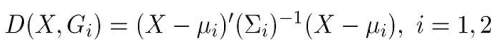判别准则：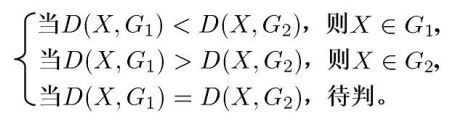（1）等方差阵：直线判别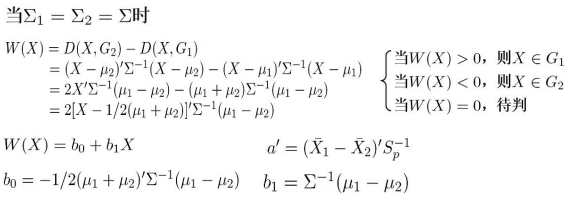（2）异方差阵：曲线判别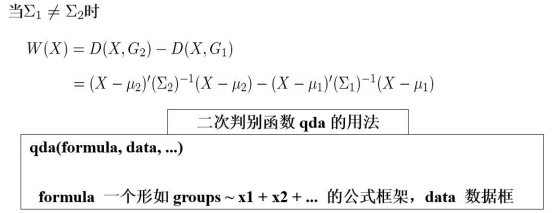1.2.2 多总体距离判别
与两总体距离判别类似：

首先假定k个类别样本分属k个正态总体；
然后基于马氏距离，依次建立建立判别函数和判别规则；
基于样本信息，估计判别规则中的未知参数；
带入未知样本信息，判别其类别。

（1）协方差矩阵相同：线性判别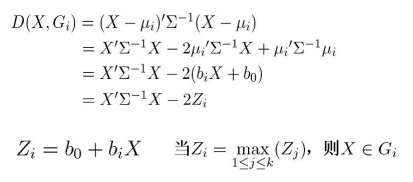（2）协方差矩阵不同：非线性判别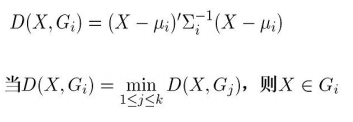1.3 Fisher 判别法
1.3.1 Fisher 判别法原理
在距离判别法中，向量X的维数较高：

均值、协方差估计中待估参数较多；
导致判别规则中存在较大的误差。

Fisher在1936年提出了Fisher判别法：

把高维空间的点向低维空间投影；
先投影到一维空间上，如果判别效果不理想，再投影到另一条直线上（从而构成二维空间）；
以此类推，每个投影可以建立一个判别函数。

即，利用一条过原点的判别函数，使得不同类别在判别函数上投影的距离尽可能大，而同一类别的距离尽可能小。
1.3.2 Fisher 判别法步骤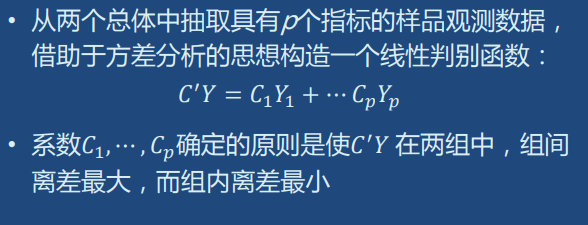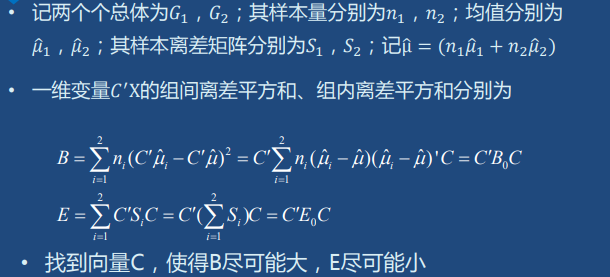1.4 Bayes 判别法
1.4.1 Bayes 判别法概念
Fisher判别缺陷：

判别方法与各总体出现的概率无关
判别方法与错判后造成的损失无关

Bayes判别准则：

以个体归属于某类的概率（判别值）最大或错判总平均损失最小为标准。

1.4.2 概率判别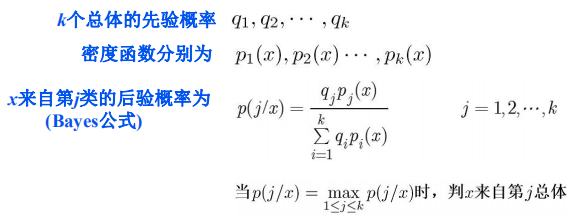1.4.3 损失判别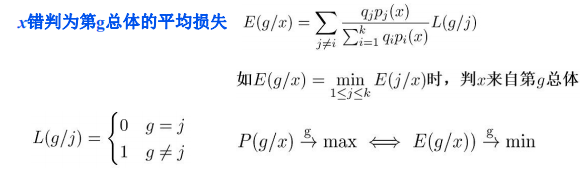1.5 几种判别方法总结
（1）常用的判别方法有Fisher判别、距离判别、贝叶斯判别等，每个方法根据 其出发点不同各有其特点。
（2） Fisher类判别对判别变量的分布类型并无要求，而Bayes类判别要变量的分 布类型。因此，Fisher类判别较Bayes类判别简单一些。
（3）当两个总体时，若它们的协方差矩阵相同，则距离判别和Fisher判别等价。 当变量服从正态分布时，它们还和Bayes判别等价。
1.6 Fisher判别R语言操作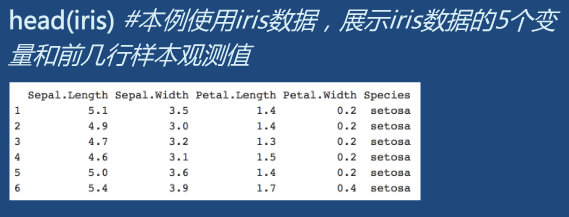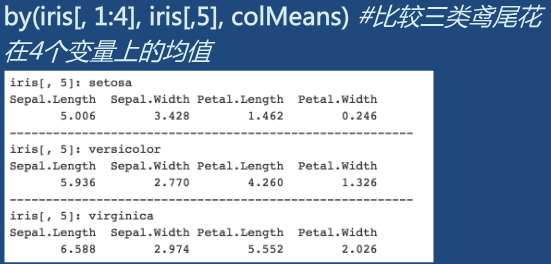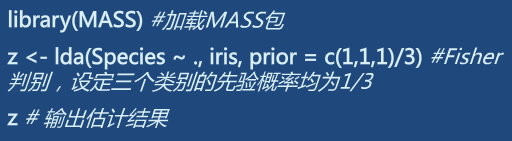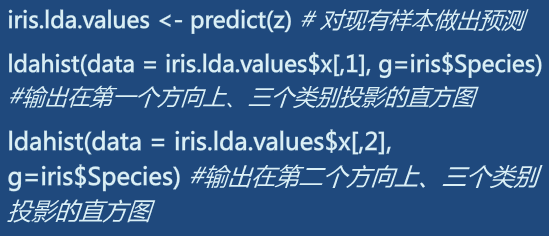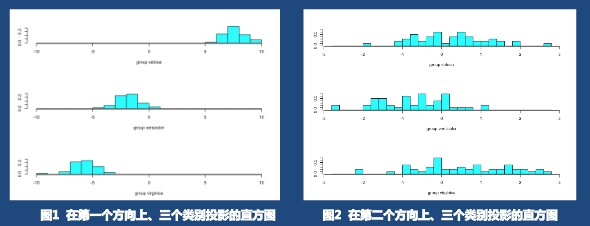2 logistic回归
2.1 logistic回归模型设定
考虑因变量y有两个取值的情况，用服从两点分布的随机变量刻画：

因变量y的期望，也就是y=1的概率只能在0和1之间取值；
对模型中的参数添加了限制，给后续的参数估计带来困难；
对因变量的期望做某个单调的变换，使得模型系数可以自由的取值

Logit变换：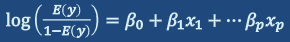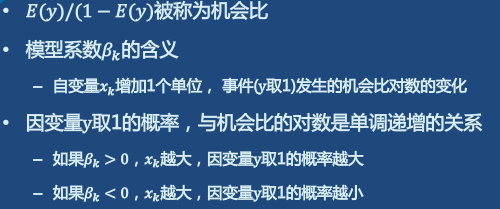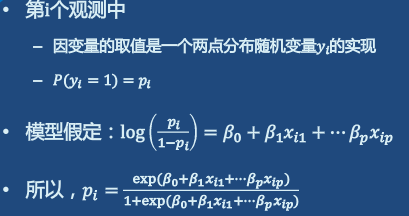Logistic 函数形似"S"，是Sigmoid函数的典型代表，它将z值转化为一个接近0或1的y值，并且其输出值在z=0附近变化很陡。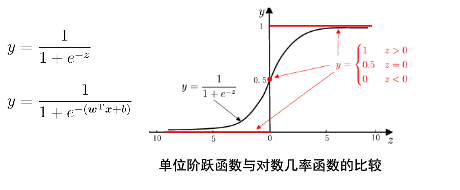2.2 Logistic回归模型系数估计2.3 Logistic回归模型系数的推断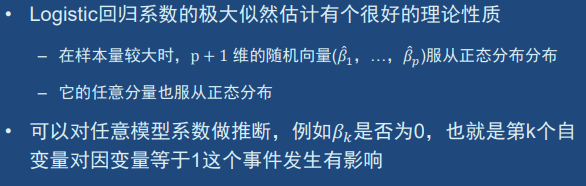类似回归模型，我们可以从全模型出发，依次删去不显著的自变量，找到一个最终模型
当有了新的观测

基于其自变量的取值，估计出这个观测对应因变量为1的概率
进一步基于这个概率，对因变量做出预测，也就是对这个观测做出分类

2.3 Logistic回归R语言操作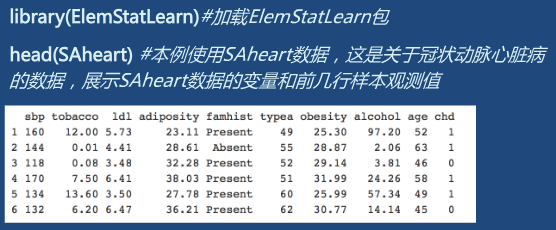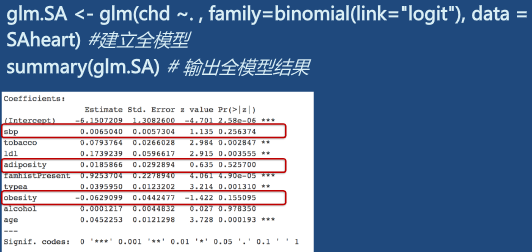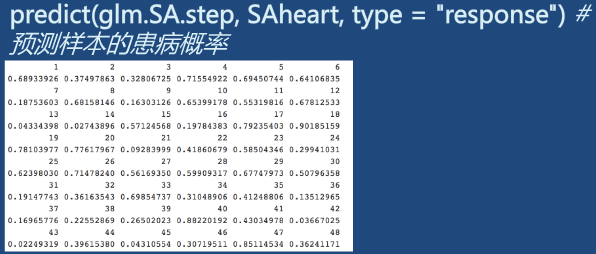相关笔记：

Python相关实用技巧01：安装Python库超实用方法，轻松告别失败！
Python相关实用技巧02：Python2和Python3的区别
Python相关实用技巧03：14个对数据科学最有用的Python库
Python相关实用技巧04：网络爬虫之Scrapy框架及案例分析
Python相关实用技巧05：yield关键字的使用
Scrapy爬虫小技巧02：HTTP status code is not handled or not allowed的解决方法
数据分析学习总结笔记01：情感分析
数据分析学习总结笔记02：聚类分析及其R语言实现
数据分析学习总结笔记03：数据降维经典方法
数据分析学习总结笔记04：异常值处理
数据分析学习总结笔记05：缺失值分析及处理
数据分析学习总结笔记06：T检验的原理和步骤
数据分析学习总结笔记07：方差分析
数据分析学习总结笔记07：回归分析概述
数据分析学习总结笔记08：数据分类典型方法及其R语言实现
数据分析学习总结笔记09：文本分析
数据分析学习总结笔记10：网络分析

本文主要根据个人学习（机器学习MOOC、有用的统计学MOOC、多元统计分析MOOC），并搜集部分网络上的优质资源总结而成，如有不足之处敬请谅解，欢迎批评指正、交流学习！

`
展开全文• 简单相关系数的代码实现 1.XY都是随机变量，地位对称 2.相关系数只反映两变量之间线性相关的程度，不能说明其非线性相关关系。 3.虽能度量相关关系，但是不能度量变量间的因果关系 公式 library('charlatan') # ...
• 典型相关分析matlab实现代码 Python - 100天从新手到大师 作者-chars Python应用领域和就业形势分析 简单的说，Python是一个“优雅”、“明确”、“简单”的编程语言。 学习曲线低，非专业人士也能上手 开源系统，...
• 典型相关分析matlab实现代码 Python - 100天从新手到大师 by 骆昊 Python应用领域和就业形势分析 简单的说，Python是一个“优雅”、“明确”、“简单”的编程语言。 学习曲线低，适合非专业人士 开源系统，拥有强大......